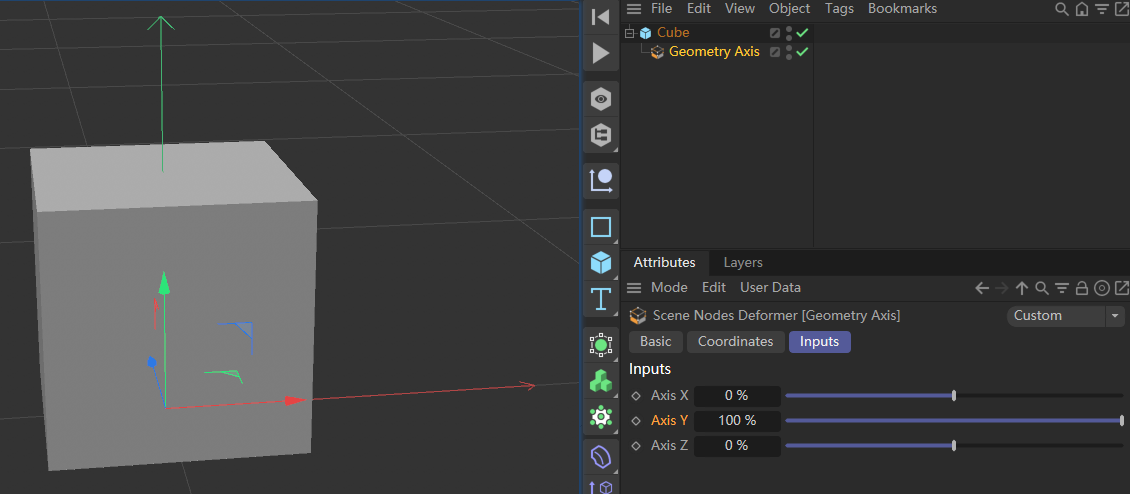# Geometry Axis asset node and LoadAssets

My script works and I got what I want, but there are two problems.

• `maxon.AssetManagerInterface.LoadAssets` always return False event the asset loaded successfully.

• I set the parameters of Geometry Axis by find the param's name. Is there a better way to change the parameters?

``````import maxon, c4d

def loadGeometryAxis(x=0, y=0, z=0, parent: c4d.BaseList2D = None):
repository = maxon.AssetInterface.GetUserPrefsRepository()
if not repository:
raise RuntimeError("Could not access the user preferences repository.")
# Geometry Axis asset id
assetid = maxon.Id("net.maxon.neutron.asset.geo.geometryaxis")
sceneNodesHook = doc.FindSceneHook(c4d.SCENENODES_IDS_SCENEHOOK_ID)
if not sceneNodesHook:
raise RuntimeError("Could not retrieve Scene Nodes scene hook.")
sceneNodesHook.Message(maxon.neutron.MSG_CREATE_IF_REQUIRED)
sceneNodes = sceneNodesHook.GetNimbusRef(maxon.neutron.NODESPACE)
if not sceneNodes:
raise RuntimeError("Could not retrieve Scene Nodes graph model.")
graph = sceneNodes.GetGraph()
# What's wrong?
...
obj = doc.GetFirstObject()
# Is there is a better way to change the parameters?
for bc, descid, _ in obj.GetDescription(c4d.DESCFLAGS_DESC_0):
name = bc[c4d.DESC_NAME]
if name == "Axis X":
obj[descid] = x
if name == "Axis Y":
obj[descid] = y
if name == "Axis Z":
obj[descid] = z
if parent is not None:
obj.InsertUnderLast(parent)

if __name__ == "__main__":
cube = c4d.BaseObject(c4d.Ocube)
doc.InsertObject(cube)

``````Hi,

In this case, the asset loaded is a capsule. For capsule, the return value can be false. This looks like a bug in our API. One remark about your code, as you want to load a capsule, you do not need to pass a graphModelRef to the LoadAssets function.

How to access or change the parameter value, is a good question. To access the data, you must use the descID as you understood but the descID of capsules are exceptionally long. It seems those descID are based on either the path of the port or the ID of the port.
I asked the devs but for now, it seems that the best attribut to identify the parameter is its name. Of course, this is bad as the name will change from languages to languages.

Cheers,
Manuel

@manuel
Thank you very much to answer my confusion. If I do not need to pass a graphModelRef to the LoadAssets function, my code can be simplified a lot. Can it be okay to identify the parameter by the index of iterating the capsule's description. Since the capsule is newly created, and there're no extra parameters, so it seems the index is fixed.

First, get the index by following code.

``````for i, (bc, descid, _) in enumerate(capsule.GetDescription(c4d.DESCFLAGS_DESC_0)):
name = bc[c4d.DESC_NAME]
if name == "Axis X":
print(i) # 59
if name == "Axis Y":
print(i) # 60
if name == "Axis Z":
print(i) # 61
``````

Then, the total function def:

``````def loadGeometryAxis(x=0, y=0, z=0, parent: c4d.BaseObject = None):
repository = maxon.AssetInterface.GetUserPrefsRepository()
if not repository:
raise RuntimeError("Could not access the user preferences repository.")
# Geometry Axis asset id
assetid = maxon.Id("net.maxon.neutron.asset.geo.geometryaxis")